# nLab Born rule

Contents

### Context

#### Probability theory

measure theory

probability theory

# Contents

## Idea

The Born rule is the core statement of coordination in the foundations of quantum physics:

In its version for pure states the Born rule says that

then the probability of observing the given eigenstate in a quantum system which is in state $\psi$ equals (in bra-ket notation):

$\frac{ \langle \psi \vert P \vert \psi \rangle }{ \langle \psi \vert \psi \rangle } \,.$

In particular, if $W$ is an orthonormal basis for the Hilbert space of states associated with a quantum measurement-procedure, then the probability of measuring the result $w$ on a system in state $\left\vert \psi \right\rangle$ is

$\frac{ \langle \psi \vert w \rangle \langle w \vert \psi \rangle }{ \langle \psi \vert \psi \rangle } \;\;=\;\; \frac{ \big\vert \langle w \vert \psi \rangle \big\vert^2 }{ \langle \psi \vert \psi \rangle }$

and so if the state is normalized to begin with ($\langle \psi \vert \psi \rangle$ = 1 ), then the probability is

$P_\psi(w) \;=\; \big\vert \langle w \vert \psi \rangle \big\vert^2 \,.$

Essentially in this form the rule was first formulated by Born 1926b p 805.

### Historical origins

The Born rule is named in honor of

where it appears (though disregarding the norm symbol) as a brief footnote-added-in-proof: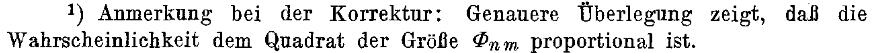and its expanded version

which provides the mathematical details (p. 805):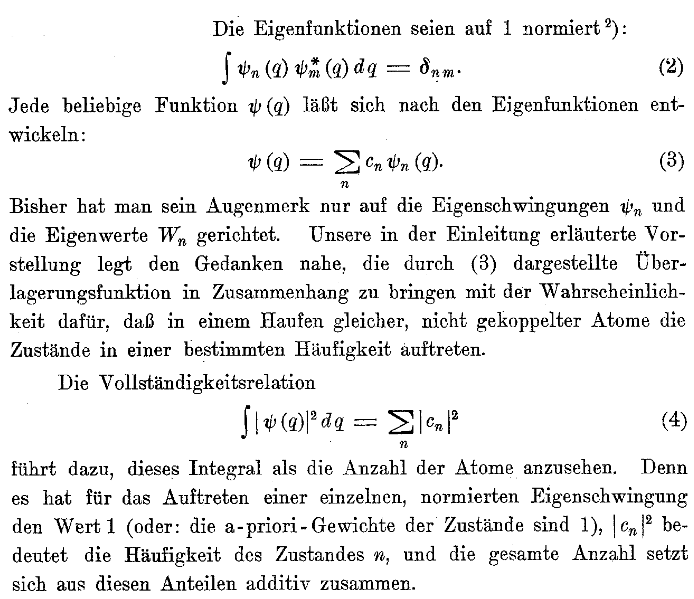and finally in the followup

which makes the interpretation more explicit (p. 171):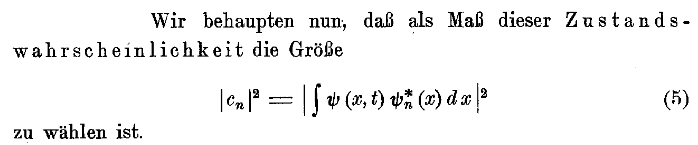The generalization of this idea to probability densities for continuous observables is due to Wolfgang Pauli, as first recounted in: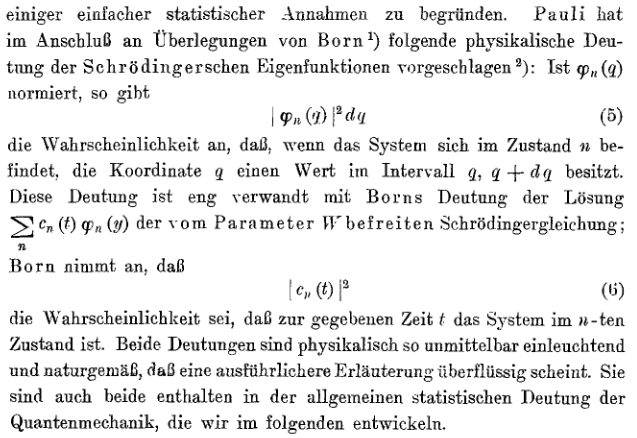and then by Pauli himself, again in a footnote: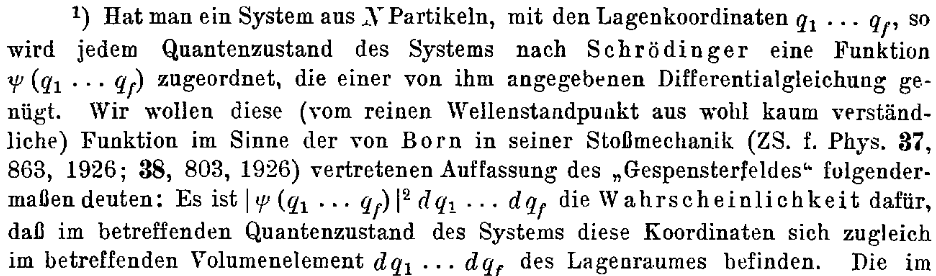Early review of the Born-Pauli rule:

where it is once again a footnote: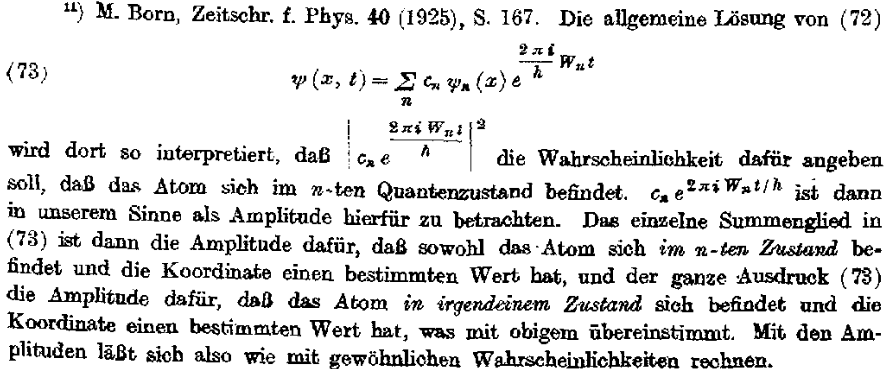and in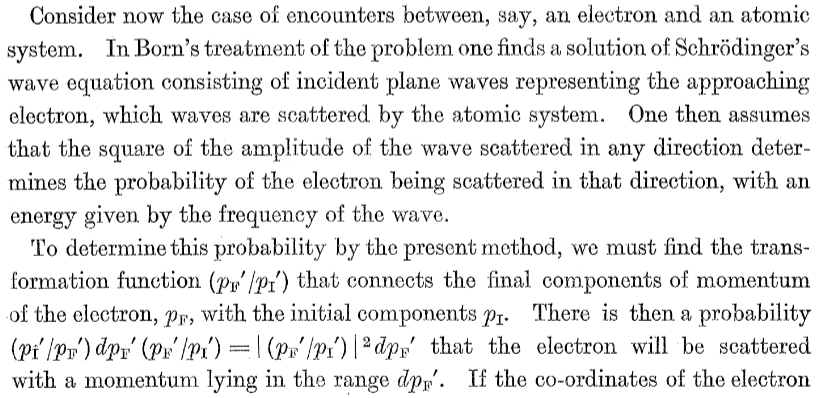The full recognition and amplification of the Born rule as a pillar of quantum physics making it a probabilistic theory (cf. quantum probability) is (maybe besides Jordan 1927) due to: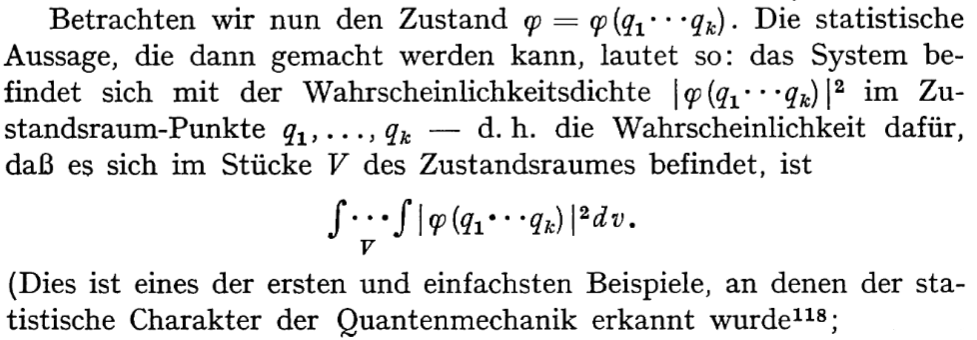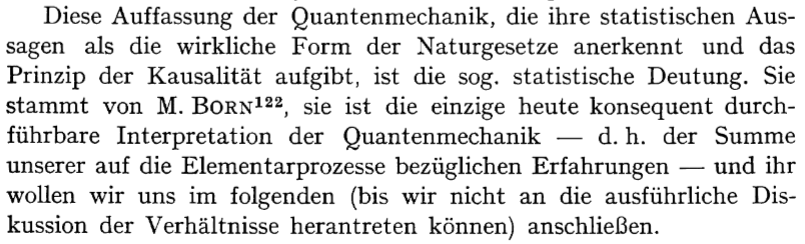Historical survey:

• Jagdish Mehra, Helmut Rechenberg, The Probability Interpretation and the Statistical Transformation Theory, the Physical Interpretation, and the Empirical and Mathematical Foundations of Quantum Mechanics 1926-1932, Part 1 in: The Historical Development of Quantum Theory. Volume 6: The Completion of Quantum Mechanics, 1926-1941, Springer (2001) [ISBN:978-0-387-98971-6]

Review: